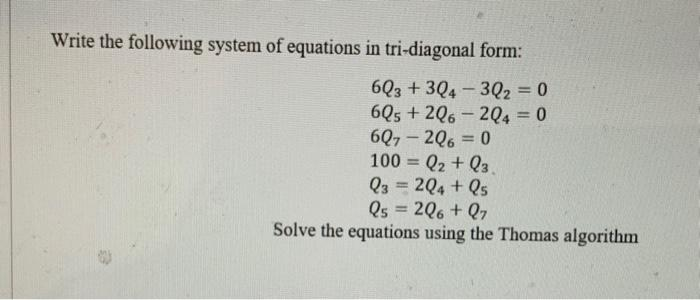# (Solved): USE MATLAB PLEASE Write the following system of equations in tri-diagonal form: 6Q3 +3Q4-3Q = 0 6 ...

Write the following system of equations in tri-diagonal form: 6Q3 +3Q4-3Q₂ = 0 6Q5+2Q62Q4 = 0 6Q7 – 2Q6 = 0 100 = Q₂ + Q3 Q3 = 2Q4 + Q5 Q5 = 2Q6+ Q7 Solve the equations using the Thomas algorithmWrite the following system of equations in tri-diagonal form: Solve the equations using the Thomas algorithm

We have an Answer from Expert